#Function Repository Resource:

# Loess

Smooth noisy data using local regression

Contributed by: Jon McLoone
 ResourceFunction["Loess"][data, bandwidth, x] finds the interpolation of data at x by using local regression smoothing of bandwidth data points. ResourceFunction["Loess"][data, Scaled[bandwidth], x] finds the interpolation of data at x by using local regression smoothing of bandwidth fraction of data points. ResourceFunction["Loess"][data,bandwidth,{x1,x2,…}] finds the interpolation of data at each xi by using local regression smoothing of bandwidth data points.

## Details and Options

Data can be any dimensions.

## Examples

### Basic Examples

Loess is useful when data is noisy but has an underlying trend:

 In:=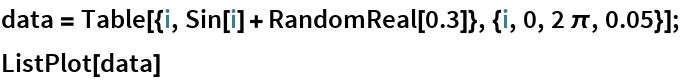Out=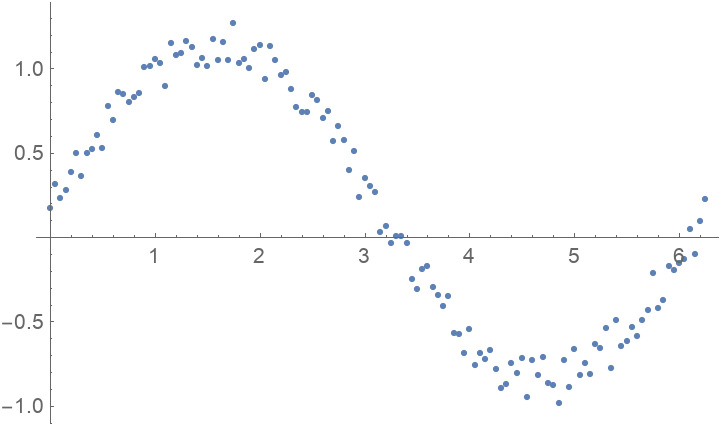Find an estimated value for the data at x=2 using the nearest 12 data points:

 In:=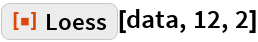Out=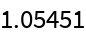Find an estimated value for the data at x=2 using the nearest 10% of the data:

 In:=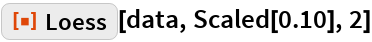Out=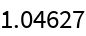In:=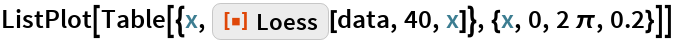Out=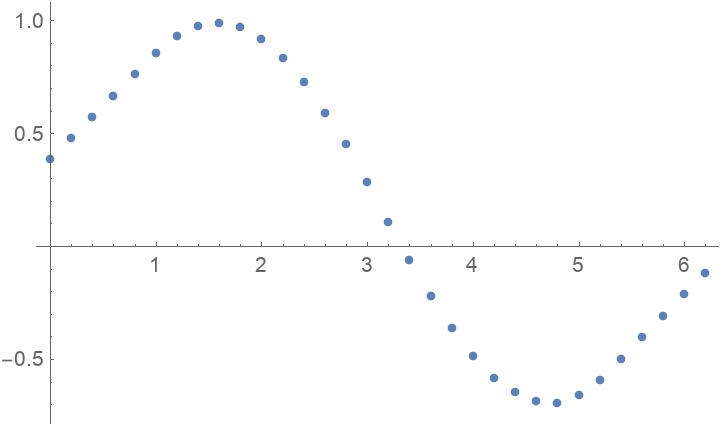### Scope

Loess can handle higher-dimensional data:

 In:=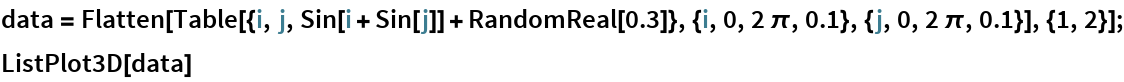Out=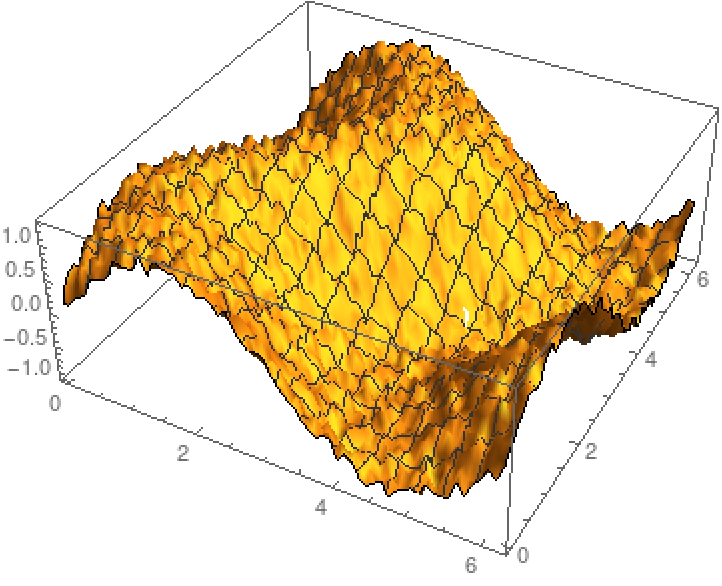In:=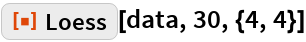Out=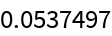In:=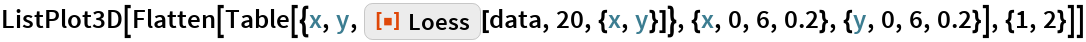Out=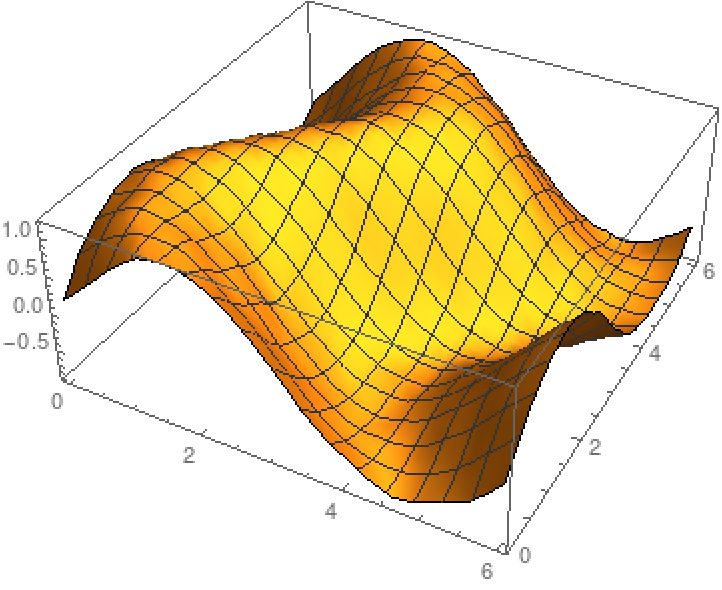### Options

By default, Loess fits straight lines to subsets of data, but you can increase the interpolation order to capture different detail:

 In:=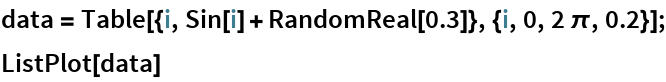Out=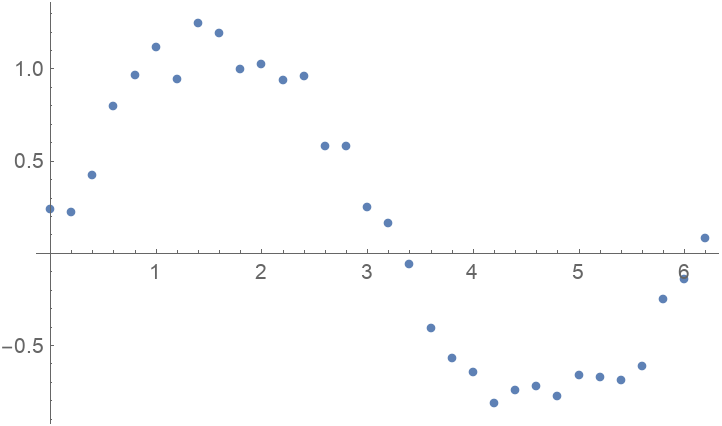In:=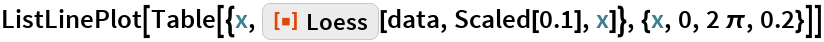Out=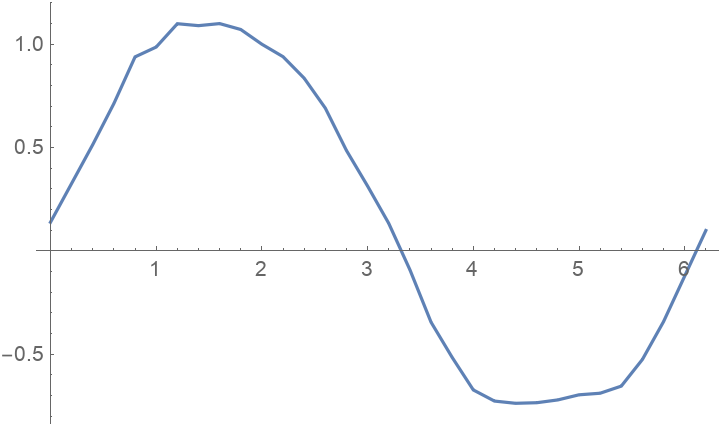In:=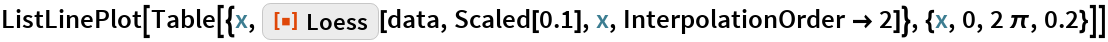Out=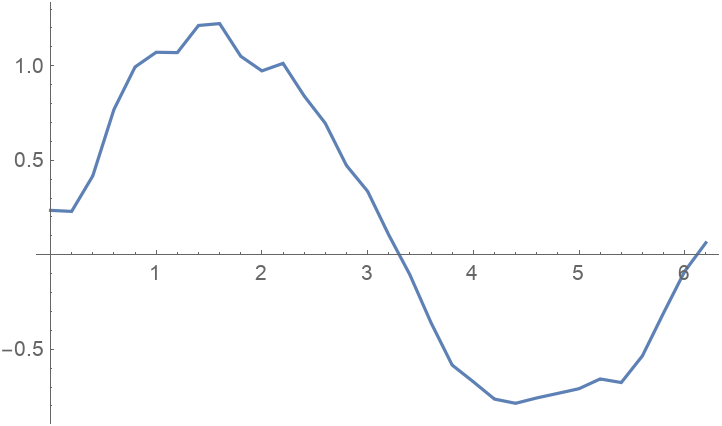Loess fitting involves weighting the local data. Typically, points further from the estimated point are given less weight:

 In:=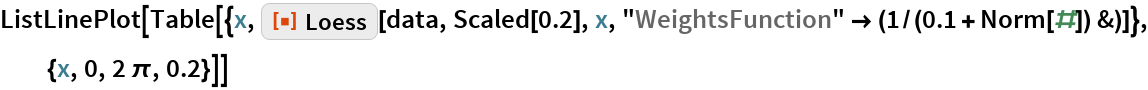Out=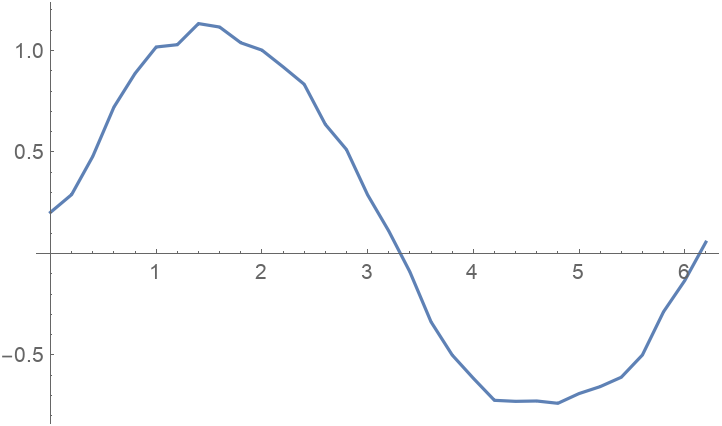### Possible Issues

If you intend to use Loess to predict many values from the same data, then it is more efficient to find all values in a single request:

 In:=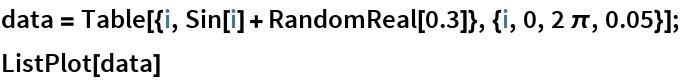Out=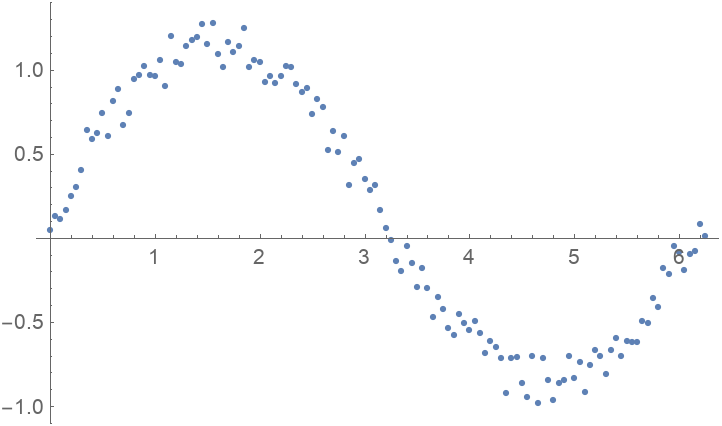This method:

 In:=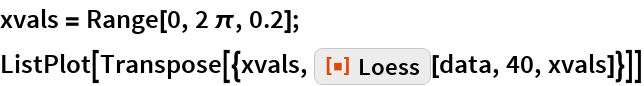Out=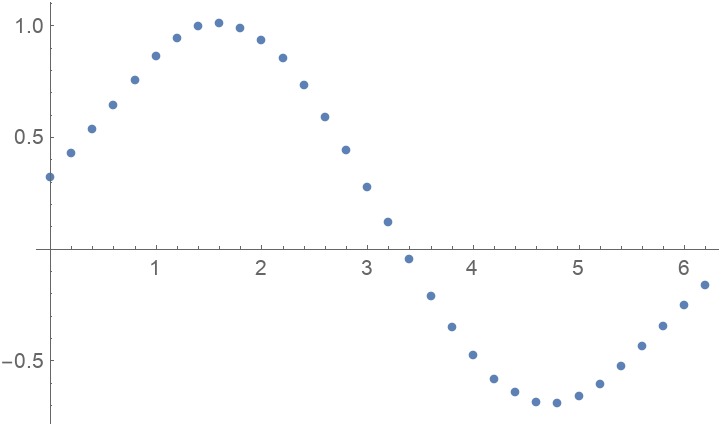Is faster than this method:

 In:=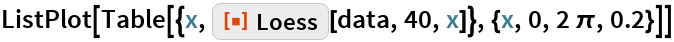Out=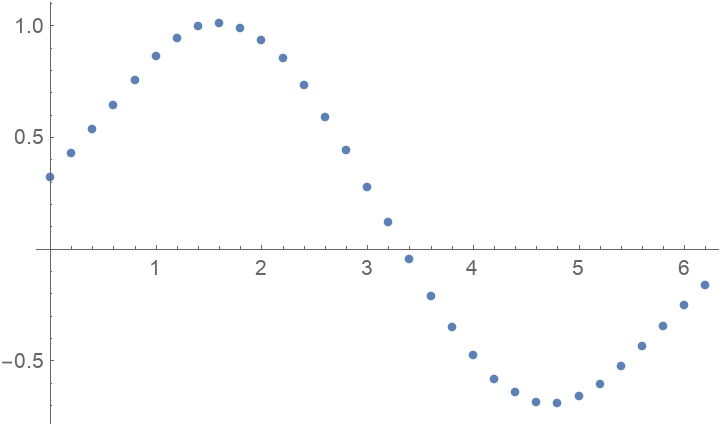## Requirements

Wolfram Language 11.3 (March 2018) or above